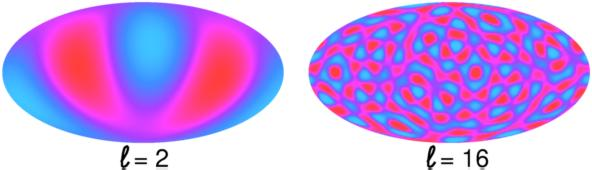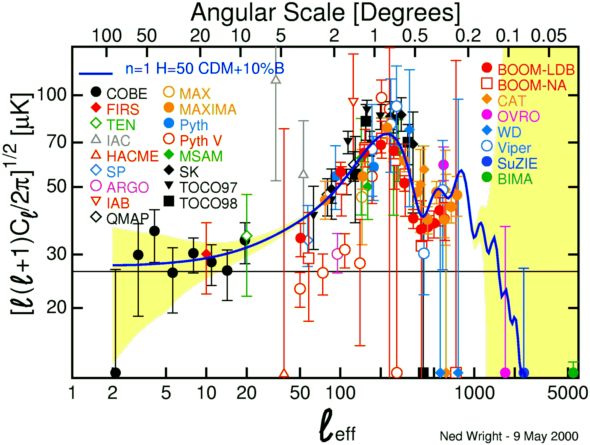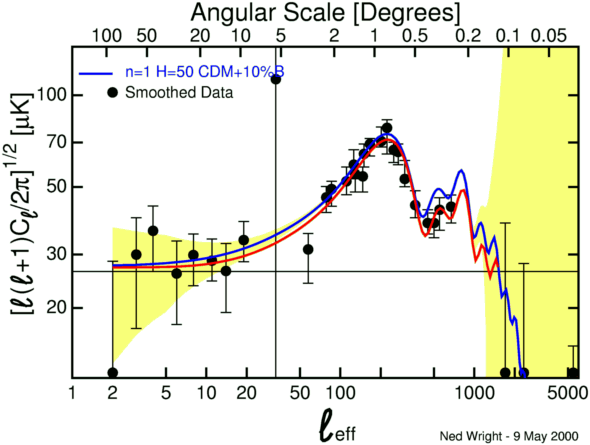# Cosmic Microwave Background Anisotropy

The angular power spectrum of the anisotropy of the CMB contains information about the formation of the Universe and its current contents. This angular power spectrum is a plot of how much the temperature varies from point to point on the sky (the y-axis variable) vs. the angular frequency ell (the x-axis variable). Ell=10 means that there are ten cycles in the fluctuation around the whole sky, while ell=100 means that there are 100 cycles around the sky.The image above shows an all-sky map with only ell=2 power on the left, and another map with only ell=16 power on the right.

Many groups are trying the measure the angular power spectrum, and this data is expected to answer fundamental questions about the nature of the Universe. So it gets into the newspapers often, from the COBE results in April 1992 to the BOOMERanG results in April 2000. The current status of microwave background anisotropy measurements in my compilation from the literature has 95 data points from 25 experiments. These are all plotted in the figure below:This figure looks rather messy because many of the experimental groups have been attacking small parts of the spectrum with limited signal-to-noise.

But if we bin and average the data points using a running weighted median we get the figure below which agrees well with the standard CDM model!The red curve is the model from Figure 1 of Tegmark & Zaldarriaga's interpretation of the BOOMERanG results, with Ho = 100h = 52 km/sec/Mpc, baryonic density OmegaB h2 = 0.03, CDM density OmegaCDM h2 = 0.19, and Omegalambda = 0.19. I changed the spectral index of the power law primordial power spectrum to n = 0.95 instead of n = 1. It turns out these parameters were a typographical error in this paper, but the fit is still quite good.

The yellow band in these figures is the expected noise from the MAP mission which is scheduled for launch in 2001. By doing an all-sky measurement, MAP will provide the best possible anisotropy measurements in the angular frequency range ell < 400, and will thus definitely clean up the messy region between ell = 20 and ell = 100 in the current data. For angular frequencies from 100 < ell < 250 there is already a large amount of ground-based and balloon-borne data, with more to come, but MAP will provide data with much improved sensitivity out to ell = 1000. This will provide an independent way to measure the cosmological constant which has been suggested by observations of supernovae. Even though the width of the yellow band is invisible on the figure for 400 < ell < 1000, MAP will in fact be radiometer noise limited in this band. The European space mission Planck, to be launched in 2007, will provide much better data than MAP over the range of angular frequencies 600 < ell < 2000.